Search:

# The general organic formulas

## Linear alkanesThe number of hydrogen atoms is the double of the number of carbon atoms + 2

Here are the changes that may occur comparing to linear alkanes:

## RamificationRamification: No modification

## Double bond C=C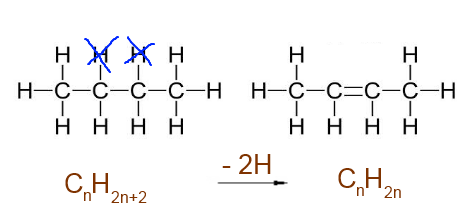Double bond C=C : -2 H atoms

## Triple bond C$\equiv$CTriple bond C$\equiv$C : -4 H atoms

## CycleCycle : -2 H atoms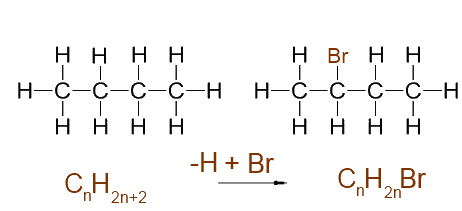One added halogen : - 1 H atom+ 1 halogen atom

## Oxygen interposed between C and H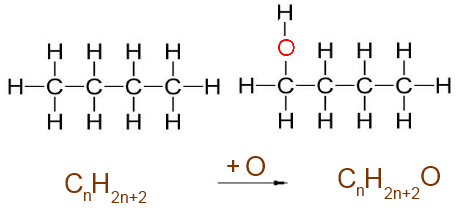Oxygen interposed between C and H : + 1 O atom

## Oxygen interposed between C and COxygen interposed between C and C : + 1 O atom.

## Double bond C=O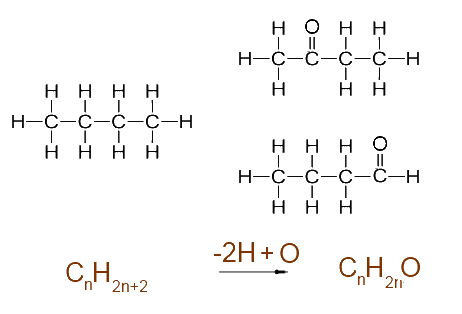Double bond C=O : -2 H atoms + 1 O atom

## Nitrogen interposed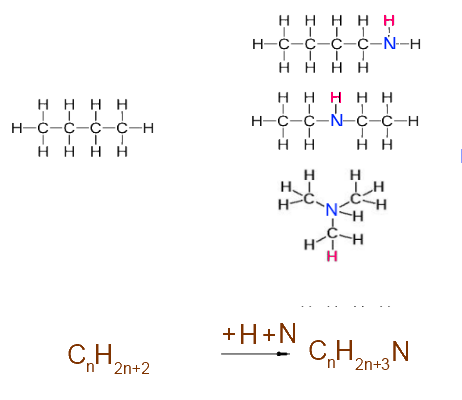Nitrogen interposed : +1 H atom + 1 N atom

## Example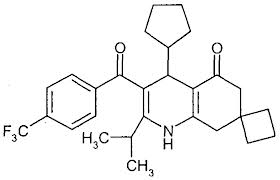The molecule has 28 C atoms, The corresponding linear alkane would have the formula $C_{28}H_{58}$ 5 cycles:→ -10H 5 C=C:→ -10H 2 C=O→ -4H+2O 1 N:→ +1H+N 3 F:→ -3H+3F ..so the molecular formula is: $C_{28}H_{32}O_2NF_3$